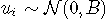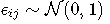# 模型背景

## 潜类别混合模型

• X（t） 和Z（t） 是协变量的向量（Z（t） ；
• β 是固定效应（即总体均值效应）；
• ui 是随机效应（即个体效应）；它们根据具有协方差矩阵B 的零均值多元正态分布进行分布；
• （wi（t）） 是高斯过程，可以添加到模型中以来放宽对象内部相关结构。

• tij 是主题i 和场合j 的测量时间；
• ϵij 是一个独立的零均值高斯误差；
• H 是链接函数，可将潜过程转换为比例和度量。

• 线性变换：这简化为线性混合模型（2个参数）
• Beta累积分布族重新调整（4个参数）

# 示例

## 考虑的模型：## 估计不同连续链接函数的模型H

### 线性链接函数

``lcmm(CESD ~ age65*male, random=~ age65 #链接=线性``

``hlme(CESD ~ age65*male, random=~ age65 #链接=线性``

``````loglik
 -7056.652``````

# R语言建立和可视化混合效应模型mixed effect model

``lcmm( random=~ age65, link='beta')``

``lcmm(random=~ age65, subject='ID', link='splines')``

### 随时关注您喜欢的主题

``lcmm(link='5-quant-splines')``

## 选择最佳模型

AIC（每个模型的输出中都有UACV）：

``````plot(mli, which="linkfunction",xlab="潜过程")
legend(x="topleft", legend=c("线性", "beta","样条曲线 (5个等距结点)","样条曲线(5个分位数结点)"))``````

# 我们看到2个样条曲线转换非常接近。线性模型似乎不合适，如线性曲线和样条曲线之间的差值所示。Beta转换仅在潜过程的高值时才与样条曲线不同。变换的置信带可以通过蒙特卡洛方法获得：

``````predict(mspl5q,ndraws=2000)
legend(legend=c("95% 置信带","分位数样条"),lty=c(2,NA))``````

# 注意，该模型成为累积概率混合模型。这里是一个使用HIER变量(4级)的例子，因为考虑到0-52的范围(例如52个阈值参数)，CESD的阈值链接函数会涉及太多参数。

## 用离散链接函数H估计模型

`` lcmm(HIER ~ age65*male, link='thresholds')``

### 概要

``predict(msp, newdata=datnew, var.time="age"``

``````plot(women,xlab="年龄")
legend(legend=c("女性","男性", "95% 置信区间", "95% 置信区间"))``````

### 拟合优度1：残差图

``plot( var.time="age65", xlab="(年龄-65)/10", break.times=8, ylab="潜过程")``

Kaizong Ye拓端研究室（TRL）的研究员。

​非常感谢您阅读本文，如需帮助请联系我们！QQ在线咨询

15121130882

0571-63341498

## 关注有关新文章的微信公众号

This will close in 0 seconds